# Certain unified integrals associated with Bessel functions

## Abstract

A remarkably large number of integral formulas involving a variety of special functions have been developed by many authors. Very recently, Ali gave three interesting unified integrals involving the hypergeometric function ${}_{2}F_{1}$. Using Ali’s method, in this paper, we present two generalized integral formulas involving the Bessel function of the first kind ${J}_{\nu }\left(z\right)$, which are expressed in terms of the generalized (Wright) hypergeometric functions. Some interesting special cases of our main results are also considered.

MSC:33B20, 33C20, 33B15, 33C05.

## 1 Introduction and preliminaries

Integrals involving products of Gamma functions along vertical lines were first studied by Pincherle in 1888 and an extensive theory was developed by Barnes  and Mellin . Cahen  employed some of these integrals in the study of the Riemann Zeta function and other Dirichlet series. In a spirit of Mellin’s theory, some of Ramanujan’s formulas were generalized by Hardy [, p.98]. The work of Pincherle provided an impetus for the subsequent investigations of Barnes  and Mellin  on the integral representations of solutions of generalized hypergeometric series (see [, Chapter 16 and the comment on p.225]). A detailed commentary on Pincherle’s work  set against a historical backdrop is available in .

Indeed, a remarkably large number of integral formulas involving a variety of special functions have been developed by many authors (see, for example, ; for a very recent work, see also ). Recently, Garg and Mittal  obtained an interesting unified integral involving Fox H-function. Motivated by the work of Garg and Mittal , very recently, Ali  gave three interesting unified integrals involving the hypergeometric function ${}_{2}F_{1}$. Also, many integral formulas involving the Bessel function ${J}_{\nu }\left(z\right)$ (1.1) have been presented (see, e.g., [, pp.196-204]; see also [, pp.373-476]). Here, by using Ali’s method , we aim at presenting two generalized integral formulas involving the Bessel function of the first kind (1.1), which are expressed in terms of the generalized (Wright) hypergeometric functions (1.4). Some interesting special cases of our main results are also considered.

For our purpose, we begin by recalling some known functions and earlier works. The Bessel function of the first kind ${J}_{\nu }\left(z\right)$ is defined for $z\in \mathbb{C}\setminus \left\{0\right\}$ and $\nu \in \mathbb{C}$ with $\mathrm{\Re }\left(\nu \right)>-1$ by the following series (see, e.g., [, p.217, Entry 10.2.2] and [, p.40, Eq. (8)]):

${J}_{\nu }\left(z\right)=\sum _{k=0}^{\mathrm{\infty }}\frac{{\left(-1\right)}^{k}{\left(\frac{z}{2}\right)}^{\nu +2k}}{k!\mathrm{\Gamma }\left(\nu +k+1\right)}={\frac{{\left(z/2\right)}^{\nu }}{\mathrm{\Gamma }\left(1+\nu \right)}}_{0}{F}_{1}\left(\overline{\phantom{\phantom{\rule{1em}{0ex}}}}\phantom{\rule{0.2em}{0ex}};1+\nu ;-\frac{{z}^{2}}{4}\right),$
(1.1)

where ${}_{0}F_{1}$ is a confluent hypergeometric series of ${}_{p}F_{q}$ in (1.5), denotes the set of complex numbers and $\mathrm{\Gamma }\left(z\right)$ is the familiar Gamma function (see [, Section 1.1]).

An interesting further generalization of the generalized hypergeometric series ${}_{p}F_{q}$ (1.5) is due to Fox  and Wright  who studied the asymptotic expansion of the generalized (Wright) hypergeometric function defined by (see [, p.21]; see also )

${}_{p}\mathrm{\Psi }_{q}\left[\begin{array}{ll}\begin{array}{l}\left({\alpha }_{1},{A}_{1}\right),\dots ,\left({\alpha }_{p},{A}_{p}\right);\\ \left({\beta }_{1},{B}_{1}\right),\dots ,\left({\beta }_{q},{B}_{q}\right);\end{array}& z\end{array}\right]=\sum _{k=0}^{\mathrm{\infty }}\frac{{\prod }_{j=1}^{p}\mathrm{\Gamma }\left({\alpha }_{j}+{A}_{j}k\right)}{{\prod }_{j=1}^{q}\mathrm{\Gamma }\left({\beta }_{j}+{B}_{j}k\right)}\frac{{z}^{k}}{k!},$
(1.2)

where the coefficients ${A}_{1},\dots ,{A}_{p}$ and ${B}_{1},\dots ,{B}_{q}$ are positive real numbers such that

$1+\sum _{j=1}^{q}{B}_{j}-\sum _{j=1}^{p}{A}_{j}\geqq 0.$
(1.3)

A special case of (1.2) is

${}_{p}\mathrm{\Psi }_{q}\left[\begin{array}{ll}\begin{array}{l}\left({\alpha }_{1},1\right),\dots ,\left({\alpha }_{p},1\right);\\ \left({\beta }_{1},1\right),\dots ,\left({\beta }_{q},1\right);\end{array}& z\end{array}\right]={\frac{{\prod }_{j=1}^{p}\mathrm{\Gamma }\left({\alpha }_{j}\right)}{{\prod }_{j=1}^{q}\mathrm{\Gamma }\left({\beta }_{j}\right)}}_{p}{F}_{q}\left[\begin{array}{ll}\begin{array}{l}{\alpha }_{1},\dots ,{\alpha }_{p};\\ {\beta }_{1},\dots ,{\beta }_{q};\end{array}& z\end{array}\right],$
(1.4)

where ${}_{p}F_{q}$ is the generalized hypergeometric series defined by (see [, Section 1.5])

$\begin{array}{rcl}{}_{p}F_{q}\left[\begin{array}{ll}\begin{array}{l}{\alpha }_{1},\dots ,{\alpha }_{p};\\ {\beta }_{1},\dots ,{\beta }_{q};\end{array}& z\end{array}\right]& =& \sum _{n=0}^{\mathrm{\infty }}\frac{{\left({\alpha }_{1}\right)}_{n}\cdots {\left({\alpha }_{p}\right)}_{n}}{{\left({\beta }_{1}\right)}_{n}\cdots {\left({\beta }_{q}\right)}_{n}}\frac{{z}^{n}}{n!}\\ =& {}_{p}F_{q}\left({\alpha }_{1},\dots ,{\alpha }_{p};{\beta }_{1},\dots ,{\beta }_{q};z\right),\end{array}$
(1.5)

where ${\left(\lambda \right)}_{n}$ is the Pochhammer symbol defined (for $\lambda \in \mathbb{C}$) by (see [, p.2 and pp.4-6]):

$\begin{array}{rcl}{\left(\lambda \right)}_{n}:& =& \left\{\begin{array}{cc}1\hfill & \left(n=0\right),\hfill \\ \lambda \left(\lambda +1\right)\cdots \left(\lambda +n-1\right)\hfill & \left(n\in \mathbb{N}:=\left\{1,2,3,\dots \right\}\right)\hfill \end{array}\\ =& \frac{\mathrm{\Gamma }\left(\lambda +n\right)}{\mathrm{\Gamma }\left(\lambda \right)}\phantom{\rule{1em}{0ex}}\left(\lambda \in \mathbb{C}\setminus {\mathbb{Z}}_{0}^{-}\right)\end{array}$
(1.6)

and ${\mathbb{Z}}_{0}^{-}$ denotes the set of nonpositive integers.

For our present investigation, we also need to recall the following Oberhettinger’s integral formula :

${\int }_{0}^{\mathrm{\infty }}{x}^{\mu -1}{\left(x+a+\sqrt{{x}^{2}+2ax}\right)}^{-\lambda }\phantom{\rule{0.2em}{0ex}}dx=2\lambda {a}^{-\lambda }{\left(\frac{a}{2}\right)}^{\mu }\frac{\mathrm{\Gamma }\left(2\mu \right)\mathrm{\Gamma }\left(\lambda -\mu \right)}{\mathrm{\Gamma }\left(1+\lambda +\mu \right)},$
(1.7)

provided $0<\mathrm{\Re }\left(\mu \right)<\mathrm{\Re }\left(\lambda \right)$.

## 2 Main results

We establish two generalized integral formulas, which are expressed in terms of the generalized (Wright) hypergeometric functions (1.4), by inserting the Bessel function of the first kind (1.1) with suitable arguments into the integrand of (1.7).

Theorem 1 The following integral formula holds true: For $\lambda ,\mu ,\nu \in \mathbb{C}$ with $\mathrm{\Re }\left(\nu \right)>-1$, $0<\mathrm{\Re }\left(\mu \right)<\mathrm{\Re }\left(\lambda +\nu \right)$ and $x>0$,

$\begin{array}{r}{\int }_{0}^{\mathrm{\infty }}{x}^{\mu -1}{\left(x+a+\sqrt{{x}^{2}+2ax}\right)}^{-\lambda }{J}_{\nu }\left(\frac{y}{x+a+\sqrt{{x}^{2}+2ax}}\right)\phantom{\rule{0.2em}{0ex}}dx\\ \phantom{\rule{1em}{0ex}}={2}^{1-\nu -\mu }{a}^{\mu -\nu -\lambda }{y}^{\nu }\mathrm{\Gamma }\left(2\mu \right)\\ \phantom{\rule{2em}{0ex}}{\cdot }_{2}{\mathrm{\Psi }}_{3}\left[\begin{array}{ll}\begin{array}{l}\left(\lambda -\mu +\nu ,2\right),\left(1+\lambda +\nu ,2\right);\\ \left(\nu +1,1\right),\left(1+\lambda +\mu +\nu ,2\right),\left(\lambda +\nu ,2\right);\end{array}& -\frac{{y}^{2}}{4{a}^{2}}\end{array}\right].\end{array}$
(2.1)

Theorem 2 The following integral formula holds true: For $\lambda ,\mu ,\nu \in \mathbb{C}$ with $\mathrm{\Re }\left(\nu \right)>-1$, $0<\mathrm{\Re }\left(\mu \right)<\mathrm{\Re }\left(\lambda +\nu \right)$ and $x>0$,

$\begin{array}{r}{\int }_{0}^{\mathrm{\infty }}{x}^{\mu -1}{\left(x+a+\sqrt{{x}^{2}+2ax}\right)}^{-\lambda }{J}_{\nu }\left(\frac{xy}{x+a+\sqrt{{x}^{2}+2ax}}\right)\phantom{\rule{0.2em}{0ex}}dx\\ \phantom{\rule{1em}{0ex}}={2}^{1-2\nu -\mu }{a}^{\mu -\lambda }{y}^{\nu }\mathrm{\Gamma }\left(\lambda -\mu \right)\\ \phantom{\rule{2em}{0ex}}{\cdot }_{2}{\mathrm{\Psi }}_{3}\left[\begin{array}{ll}\begin{array}{l}\left(2\mu +2\nu ,4\right),\left(1+\lambda +\nu ,2\right);\\ \left(\nu +1,1\right),\left(1+\lambda +\mu +2\nu ,4\right),\left(\lambda +\nu ,2\right);\end{array}& -\frac{{y}^{2}}{16}\end{array}\right].\end{array}$
(2.2)

Proof By applying (1.1) to the integrand of (2.1) and then interchanging the order of integral sign and summation, which is verified by uniform convergence of the involved series under the given conditions, we get

$\begin{array}{r}{\int }_{0}^{\mathrm{\infty }}{x}^{\mu -1}{\left(x+a+\sqrt{{x}^{2}+2ax}\right)}^{-\lambda }{J}_{\nu }\left(\frac{y}{x+a+\sqrt{{x}^{2}+2ax}}\right)\phantom{\rule{0.2em}{0ex}}dx\\ \phantom{\rule{1em}{0ex}}=\sum _{k=0}^{\mathrm{\infty }}{\left(-1\right)}^{k}\frac{{\left(y/2\right)}^{\nu +2k}}{k!\mathrm{\Gamma }\left(\nu +k+1\right)}{\int }_{0}^{\mathrm{\infty }}{x}^{\mu -1}{\left(x+a+\sqrt{{x}^{2}+2ax}\right)}^{-\lambda -\nu -2k}\phantom{\rule{0.2em}{0ex}}dx.\end{array}$
(2.3)

In view of the conditions given in Theorem 1, since

$\mathrm{\Re }\left(\nu \right)>-1,\phantom{\rule{2em}{0ex}}0<\mathrm{\Re }\left(\mu \right)<\mathrm{\Re }\left(\lambda +\nu \right)\le \mathrm{\Re }\left(\lambda +\nu +2k\right)\phantom{\rule{1em}{0ex}}\left(k\in {\mathbb{N}}_{0}:=\mathbb{N}\cup \left\{0\right\}\right),$

we can apply the integral formula (1.7) to the integral in (2.3) and obtain the following expression:

$\begin{array}{r}{\int }_{0}^{\mathrm{\infty }}{x}^{\mu -1}{\left(x+a+\sqrt{{x}^{2}+2ax}\right)}^{-\lambda }{J}_{\nu }\left(\frac{y}{x+a+\sqrt{{x}^{2}+2ax}}\right)\phantom{\rule{0.2em}{0ex}}dx\\ \phantom{\rule{1em}{0ex}}={2}^{1-\nu -\mu }{a}^{\mu -\nu -\lambda }{y}^{\nu }\mathrm{\Gamma }\left(2\mu \right)\\ \phantom{\rule{2em}{0ex}}\cdot \sum _{k=0}^{\mathrm{\infty }}\frac{{\left(-1\right)}^{k}\mathrm{\Gamma }\left(1+\nu +\lambda +2k\right)\mathrm{\Gamma }\left(\nu +\lambda -\mu +2k\right)}{k!\mathrm{\Gamma }\left(1+\nu +k\right)\mathrm{\Gamma }\left(1+\nu +\lambda +\mu +2k\right)\mathrm{\Gamma }\left(\nu +\lambda +2k\right)}{\left(\frac{y}{2a}\right)}^{2k},\end{array}$

which, upon using (1.2), yields (2.1). This completes the proof of Theorem 2. □

It is easy to see that a similar argument as in the proof of Theorem 2 will establish the integral formula (2.2).

Remark We begin by stating the principle of confluence involved in ${}_{p}F_{q}$:

$\begin{array}{r}{}_{p-1}F_{q}\left({\alpha }_{1},\dots ,{\alpha }_{p-1};{\beta }_{1},\dots ,{\beta }_{q};z\right)\\ \phantom{\rule{1em}{0ex}}={\underset{|{\alpha }_{p}|\to \mathrm{\infty }}{lim}}_{p}{F}_{q}\left({\alpha }_{1},\dots ,{\alpha }_{p};{\beta }_{1},\dots ,{\beta }_{q};\frac{z}{{\alpha }_{p}}\right)\phantom{\rule{1em}{0ex}}\left(n\in \mathbb{N}\right).\end{array}$
(2.4)

In view of this principle of confluence (2.4), for example, replacing y by $y/b$ at the second integral of Ali’s work [, Eq. (2.2)] and taking the limit $|b|\to \mathrm{\infty }$ on each side of the resulting identity, we obtain

$\begin{array}{r}{\int }_{0}^{\mathrm{\infty }}{x}^{\lambda -1}\left(x+a+\sqrt{{x}^{2}+2ax}\right)^{-\nu }{}_{1}{F}_{1}\left(a;c;\frac{y}{x+a+\sqrt{{x}^{2}+2ax}}\right)\phantom{\rule{0.2em}{0ex}}dx\\ \phantom{\rule{1em}{0ex}}={\frac{{2}^{1-\lambda }\nu \mathrm{\Gamma }\left(2\lambda \right){a}^{\lambda -\nu }\mathrm{\Gamma }\left(\nu -\lambda \right)}{\mathrm{\Gamma }\left(\nu +\lambda +1\right)}}_{3}{F}_{3}\left[\begin{array}{ll}\begin{array}{l}a,\nu -\lambda ,\nu +1;\\ c,\nu +\lambda +1,\nu ;\end{array}& \frac{y}{a}\end{array}\right],\end{array}$
(2.5)

provided $0<\mathrm{\Re }\left(\lambda \right)<\mathrm{\Re }\left(\nu \right)$ and $|y/a|<1$. Again, let us try to reduce ${}_{1}F_{1}$ to ${}_{0}F_{1}$ in the integrand of (2.5) by using the principle of confluence (2.4). Replacing y by $y/a$ in the ${}_{1}F_{1}$ of (2.5) and letting $|a|\to \mathrm{\infty }$ in the resulting identity, we easily see that both sides reduce to zero. On the other hand, in view of the last expression of ${J}_{\nu }\left(z\right)$ in (1.1), we also see that ${}_{0}F_{1}$ cannot directly generate ${}_{2}F_{1}$ in the integrals of Ali’s main results [, p.152]. Even though, here, the authors make use of the method of Ali’s work  (see also ), we may carefully conclude that those results in both  and this paper do not seem to yield the other ones.

Next, we consider other variations of Theorem 1 and Theorem 2. In fact, we establish some integral formulas for the Bessel function ${J}_{\nu }\left(z\right)$ expressed in terms of the generalized hypergeometric function ${}_{p}F_{q}$. To do this, we recall the well-known Legendre duplication formula for the Gamma function Γ:

$\sqrt{\pi }\mathrm{\Gamma }\left(2z\right)={2}^{2z-1}\mathrm{\Gamma }\left(z\right)\mathrm{\Gamma }\left(z+\frac{1}{2}\right)\phantom{\rule{1em}{0ex}}\left(z\ne 0,-\frac{1}{2},-1,-\frac{3}{2},\dots \right),$
(2.6)

which is equivalently written in terms of the Pochhammer symbol (1.6) as follows (see, for example, [, p.6]):

${\left(\lambda \right)}_{2n}={2}^{2n}{\left(\frac{1}{2}\lambda \right)}_{n}{\left(\frac{1}{2}\lambda +\frac{1}{2}\right)}_{n}\phantom{\rule{1em}{0ex}}\left(n\in {\mathbb{N}}_{0}\right).$
(2.7)

Now we are ready to state the following two corollaries.

Corollary 1 Let the condition of Theorem  1 be satisfied and $\mu ,\lambda -\mu +\nu ,\lambda +\nu \in \mathbb{C}\setminus {\mathbb{Z}}_{0}^{-}$. Then the following integral formula holds true: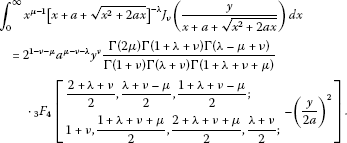(2.8)

Corollary 2 Let the condition of Theorem  2 be satisfied and $\mu +\nu ,\lambda +\nu ,\lambda -\nu \in \mathbb{C}\setminus {\mathbb{Z}}_{0}^{-}$. Then the following integral formula holds true: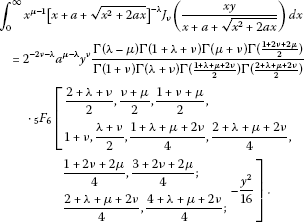(2.9)

Proof By writing the right-hand side of Eq. (2.1) in the original summation and applying (2.7) to the resulting summation, after a little simplification, we find that, when the last resulting summation is expressed in terms of ${}_{p}F_{q}$ in (1.5), this completes the proof of Corollary 1. A similar argument as in the proof of Corollary 1 will establish the integral formula (2.9). □

## 3 Special cases

In this section, we derive certain new integral formulas for the cosine and sine functions involving in the integrands of (2.1) and (2.2). To do this, we recall the following known formula (see, for example, [, p.79, Eq. (15)]):

${J}_{-1/2}\left(z\right)=\sqrt{\frac{2}{\pi z}}cosz.$
(3.1)

By applying the expression in (3.1) to (2.1), (2.2), (2.8) and (2.9), we obtain four integral formulas in Corollaries 3, 4, 5 and 6, respectively.

Corollary 3 The following integral formula holds true: For $\lambda ,\mu \in \mathbb{C}$ with $0<\mathrm{\Re }\left(\mu \right)<\mathrm{\Re }\left(\lambda \right)$ and $x>0$,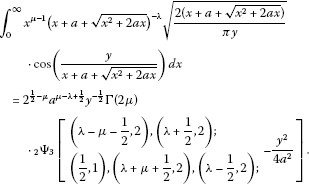(3.2)

Corollary 4 The following integral formula holds true: For $\lambda ,\mu \in \mathbb{C}$ with $0<\mathrm{\Re }\left(\mu \right)<\mathrm{\Re }\left(\lambda \right)$ and $x>0$,

$\begin{array}{r}{\int }_{0}^{\mathrm{\infty }}{x}^{\mu -1}{\left(x+a+\sqrt{{x}^{2}+2ax}\right)}^{-\lambda }\sqrt{\frac{2\left(x+a+\sqrt{{x}^{2}+2ax}\right)}{\pi xy}}\\ \phantom{\rule{2em}{0ex}}\cdot cos\left(\frac{xy}{x+a+\sqrt{{x}^{2}+2ax}}\right)\phantom{\rule{0.2em}{0ex}}dx\\ \phantom{\rule{1em}{0ex}}={2}^{2-\mu }{a}^{\mu -\lambda }{y}^{-\frac{1}{2}}\mathrm{\Gamma }\left(\lambda -\mu \right)\\ \phantom{\rule{2em}{0ex}}{\cdot }_{2}{\mathrm{\Psi }}_{3}\left[\begin{array}{ll}\begin{array}{l}\left(2\mu -1,4\right),\left(\lambda +\frac{1}{2},2\right);\\ \left(\frac{1}{2},1\right),\left(\lambda +\mu ,4\right),\left(\lambda -\frac{1}{2},2\right);\end{array}& -\frac{{y}^{2}}{16}\end{array}\right].\end{array}$
(3.3)

If we employ the same method as in getting (2.8) and (2.9) to (3.2) and (3.3), we obtain the following two corollaries.

Corollary 5 Let the condition of Corollary  3 be satisfied and $\lambda -\mu ,\lambda ,\mu \in \mathbb{C}\setminus {\mathbb{Z}}_{0}^{-}$. Then the following integral formula holds true: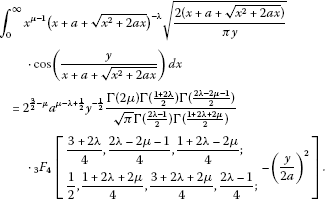(3.4)

Corollary 6 Let the condition of Corollary  4 be satisfied and $\lambda -\mu ,\lambda ,\mu \in \mathbb{C}\setminus {\mathbb{Z}}_{0}^{-}$. Then the following integral formula holds true: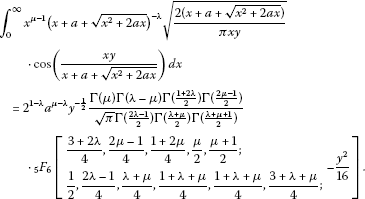(3.5)

By recalling the following formula (see, for example, [, p.79, Eq. (14)]):

${J}_{1/2}\left(z\right)=\sqrt{\frac{2}{\pi z}}sinz,$
(3.6)

and applying this formula to (2.1), (2.2), (2.8) and (2.9), we obtain four more integral formulas in Corollaries 7, 8, 9 and 10, respectively.

Corollary 7 The following integral formula holds true: For $\lambda ,\mu \in \mathbb{C}$ with $0<\mathrm{\Re }\left(\mu \right)<\mathrm{\Re }\left(\lambda \right)$ and $x>0$,

$\begin{array}{r}{\int }_{0}^{\mathrm{\infty }}{x}^{\mu -1}{\left(x+a+\sqrt{{x}^{2}+2ax}\right)}^{-\lambda }\sqrt{\frac{2\left(x+a+\sqrt{{x}^{2}+2ax}\right)}{\pi y}}\\ \phantom{\rule{2em}{0ex}}\cdot sin\left(\frac{y}{x+a+\sqrt{{x}^{2}+2ax}}\right)\phantom{\rule{0.2em}{0ex}}dx\\ \phantom{\rule{1em}{0ex}}={2}^{\frac{1}{2}-\mu }{a}^{\mu -\lambda -\frac{1}{2}}{y}^{\frac{1}{2}}\mathrm{\Gamma }\left(2\mu \right)\\ \phantom{\rule{2em}{0ex}}{\cdot }_{2}{\mathrm{\Psi }}_{3}\left[\begin{array}{ll}\begin{array}{l}\left(\lambda -\mu +\frac{1}{2},2\right),\left(\lambda +\frac{3}{2},2\right);\\ \left(\frac{3}{2},1\right),\left(\lambda +\mu +\frac{3}{2},2\right),\left(\lambda +\frac{1}{2},2\right);\end{array}& -\frac{{y}^{2}}{4{a}^{2}}\end{array}\right].\end{array}$
(3.7)

Corollary 8 The following integral formula holds true: For $\lambda ,\mu \in \mathbb{C}$ with $0<\mathrm{\Re }\left(\mu \right)<\mathrm{\Re }\left(\lambda \right)$ and $x>0$,

$\begin{array}{r}{\int }_{0}^{\mathrm{\infty }}{x}^{\mu -1}{\left(x+a+\sqrt{{x}^{2}+2ax}\right)}^{-\lambda }\sqrt{\frac{2\left(x+a+\sqrt{{x}^{2}+2ax}\right)}{\pi xy}}\\ \phantom{\rule{2em}{0ex}}\cdot sin\left(\frac{xy}{x+a+\sqrt{{x}^{2}+2ax}}\right)\phantom{\rule{0.2em}{0ex}}dx\\ \phantom{\rule{1em}{0ex}}={2}^{-\mu }{a}^{\mu -\lambda }{y}^{\frac{1}{2}}\mathrm{\Gamma }\left(\lambda -\mu \right)\\ \phantom{\rule{2em}{0ex}}{\cdot }_{2}{\mathrm{\Psi }}_{3}\left[\begin{array}{ll}\begin{array}{l}\left(2\mu +1,4\right),\left(\lambda +\frac{3}{2},2\right);\\ \left(\frac{3}{2},1\right),\left(\lambda +\mu +2,4\right),\left(\lambda +\frac{1}{2},2\right);\end{array}& -\frac{{y}^{2}}{16}\end{array}\right].\end{array}$
(3.8)

If we employ the same method as in getting (2.8) and (2.9) to (3.7) and (3.8), we obtain the following two corollaries.

Corollary 9 Let the condition of Corollary  7 be satisfied and $\lambda -\mu ,\lambda ,\mu \in \mathbb{C}\setminus {\mathbb{Z}}_{0}^{-}$. Then the following integral formula holds true: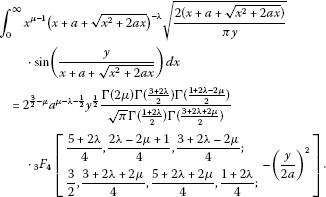(3.9)

Corollary 10 Let the condition of Corollary  8 be satisfied and $\lambda -\mu ,\nu +\mu ,\lambda ,\mu \in \mathbb{C}\setminus {\mathbb{Z}}_{0}^{-}$. Then the following integral formula holds true: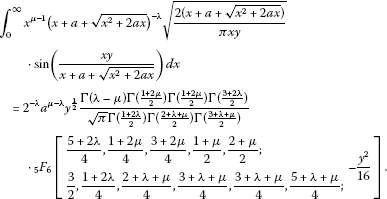(3.10)

## 4 Concluding remark

In this section, we briefly consider another variation of the results derived in the preceding sections. The Fox H-function due to Charles Fox  can be regarded as an extreme generalization of the generalized hypergeometric functions ${}_{p}F_{q}$, beyond the Meijer G-functions. Like the Meijer G-functions, the Fox H-functions turn out to be related to the Mellin-Barnes integrals and to the Mellin transforms, but in a more general way. Its asymptotic behavior and other properties of this function can be seen from the works of [23, 24] and . Further, it can be easily seen that the Bessel function of the first kind in (1.1) is a special case of the Fox H-function as follows (see [, p.2, Eq. (1.1)]):

${J}_{\nu }\left(z\right)={\left(\frac{z}{2}\right)}^{\upsilon }{H}_{0,2}^{1,0}\left[\frac{{z}^{2}}{4}|\begin{array}{c}\overline{\phantom{\phantom{\rule{2em}{0ex}}}}\\ \left(0,1\right),\left(-\upsilon ,1\right)\end{array}\right]\phantom{\rule{1em}{0ex}}\left(\mathrm{\Re }\left(\upsilon \right)>0\right).$
(4.1)

Therefore, the results presented in this paper are easily converted in terms of the Fox H-function after some suitable parametric replacement. We are also trying to find certain possible applications of those results presented here to some other research areas, for example, Srivastava and Exton  applied their integral involving the product of several Bessel functions to give an explicit expression of a generalized random walk.

## References

1. Barnes EW: A new development of the theory of the hypergeometric functions. Proc. Lond. Math. Soc. 1908, 6: 141-177.

2. Mellin HM: Abriß einer einheitlichen theorie der Gamma und der hypergeometrischen funktionen. Math. Ann. 1910, 68: 305-337. 10.1007/BF01475775

3. Cahen E:Sur la fonction $\zeta \left(\sigma \right)$ de Riemann et sur des fonctions analogues. Ann. Sci. Éc. Norm. Super. 1894, 11: 75-164.

4. Hardy GH VII. In Collected Papers. Clarendon Press, Oxford; 1979.

5. Nielsen N: Handbuch der Theorie der Gammafunktion. Teubner, Leipzig; 1906.

6. Pincherle S: Sulle funzioni ipergeometriche generalizzate. Nota I-II. In Opere Scelte-I, Unione Matematica Italiana. Ed. Cremonese, Rome; 1954:223-239.

7. Mainardi F, Pagnini G, Pincherle S: The pioneer of the Mellin-Barnes integrals. J. Comput. Appl. Math. 2003, 153: 331-342. 10.1016/S0377-0427(02)00609-X

8. Brychkov YA: Handbook of Special Functions: Derivatives, Integrals, Series and Other Formulas. CRC Press, Boca Raton; 2008.

9. Choi J, Hasanov A, Srivastava HM, Turaev M: Integral representations for Srivastava’s triple hypergeometric functions. Taiwan. J. Math. 2011, 15: 2751-2762.

10. Garg M, Mittal S: On a new unified integral. Proc. Indian Acad. Sci. Math. Sci. 2003, 114(2):99-101.

11. Ali S: On some new unified integrals. Adv. Comput. Math. Appl. 2012, 1(3):151-153.

12. Watson GN Cambridge Mathematical Library Edition. In A Treatise on the Theory of Bessel Functions. Camdridge University Press, Camdridge; 1995. Reprinted (1996)

13. Olver FWL, Lozier DW, Boisvert RF, Clark CW: NIST Handbook of Mathematical Functions. Cambridge University Press, Cambridge; 2010.

14. Srivastava HM, Choi J: Zeta and q-Zeta Functions and Associated Series and Integrals. Elsevier, Amsterdam; 2012.

15. Fox C: The asymptotic expansion of generalized hypergeometric functions. Proc. Lond. Math. Soc. 1928, 27: 389-400.

16. Wright EM: The asymptotic expansion of the generalized hypergeometric functions. J. Lond. Math. Soc. 1935, 10: 286-293.

17. Wright EM: The asymptotic expansion of integral functions defined by Taylor series. Philos. Trans. R. Soc. Lond. A 1940, 238: 423-451. 10.1098/rsta.1940.0002

18. Wright EM: The asymptotic expansion of the generalized hypergeometric function II. Proc. Lond. Math. Soc. 1940, 46: 389-408.

19. Srivastava HM, Karlsson PW: Multiple Gaussian Hypergeometric Series. Halsted, New York; 1985.

20. Rathie AK: A new generalization of generalized hypergeometric function. Matematiche 1997, LII(II):297-310.

21. Oberhettinger F: Tables of Mellin Transforms. Springer, New York; 1974.

22. Erdélyi A, Magnus W, Oberhettinger F, Tricomi FG II. In Higher Transcendental Functions. McGraw-Hill, New York; 1953.

23. Braaksma BLJ: Asymptotic expansions and analytic continuations for a class of Barnes-integrals. Compos. Math. 1964, 15: 239-341.

24. Mathai AM, Saxena RK: The H-Function with Applications in Statistics and Other Disciplines. Wiley, New York; 1978.

25. Mathai AM, Saxena RK, Haubold HJ: The H-Function: Theory and Application. Springer, New York; 2010.

26. Srivastava HM, Exton H: A generalization of the Weber-Schafheitlin integral. J. Reine Angew. Math. 1979, 309: 1-6.

## Acknowledgements

Dedicated to Professor Hari M Srivastava.

The authors should express their deepest thanks for the referees’ valuable comments and essential suggestions to improve this paper as in the present form. The first-named author was supported by Basic Science Research Program through the National Research Foundation of Korea funded by the Ministry of Education, Science and Technology (2010-0011005).

## Author information

Authors

### Corresponding author

Correspondence to Junesang Choi.

### Competing interests

The authors declare that they have no competing interests.

### Authors’ contributions

The authors have equal contributions to each part of this paper. All authors have read and approved the final manuscript.

## Rights and permissions

Reprints and Permissions

Choi, J., Agarwal, P. Certain unified integrals associated with Bessel functions. Bound Value Probl 2013, 95 (2013). https://doi.org/10.1186/1687-2770-2013-95

• Accepted:

• Published:

• DOI: https://doi.org/10.1186/1687-2770-2013-95

### Keywords

• Gamma function
• hypergeometric function ${}_{2}F_{1}$
• generalized hypergeometric function ${}_{p}F_{q}$
• generalized (Wright) hypergeometric functions ${}_{p}\mathrm{\Psi }_{q}$
• Bessel function of the first kind
• Oberhettinger’s integral formula
• Garg and Mittal’s integral formula